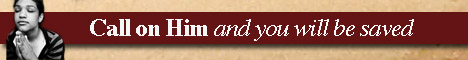Note:  Do not rely on this information. It is very old.

# Flotation

Flotation. A body will float in water if its weight be not greater than the weight of the same hulk of water. If the two weights are equal, the body will float in any position below the surface; if the body is lighter than the weight of equal bulk of water, it will rise up to the surface, and be only partially submerged. It then displaces its own weight of water. Thus, ice has a density eight-ninths that of water; eight pounds of ice have the volume of nine pounds of water. This quantity of ice will in floating displace eight pounds of liquid, which occupies eight-ninths the volume of the ice. Hence ice in floating has only one-ninth of its volume out of water - i.e. above the surface level. [Hydrostatics.]